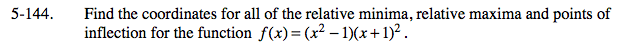### Home > CALC > Chapter 5 > Lesson 5.4.1 > Problem5-144

5-144.Max, min, and points of inflection on f(x) will occur where f '(x) = 0 or DNE or f ''(x) = 0 or DNE. But that alone is not enough to determine which is which.

Minima on f(x) happen where f '(x) changes from negative to positive.
Maxima on f(x) happen where f '(x) changes from positive to negative.
Inflection points on f(x) happen where f ''(x) changes sign.

Another way to distinguish between maxima and minima is to use the 2nd Derivative Test.